Courses

# NEET Previous Year Questions (2014-20): States of Matter Notes | EduRev

## Class 11 : NEET Previous Year Questions (2014-20): States of Matter Notes | EduRev

The document NEET Previous Year Questions (2014-20): States of Matter Notes | EduRev is a part of the Class 11 Course Chemistry 31 Years NEET Chapterwise Solved Papers.
All you need of Class 11 at this link: Class 11

Q.1. A mixture of N2 and Ar gases in a cylinder contains 7 g of N2 and 8 g of Ar. If the total pressure of the mixture of the gases in the cylinder is 27 bar, the partial pressure of N2 is:     (2020)
[Use atomic masses (in g mol-1): N = 14, Ar = 40]

(a) 15 bar
(b) 18 bar
(c) 9 bar
(d) 12 bar
Ans:
a
According to Dalton’s law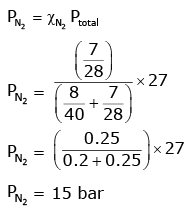Q.2. A gas at 350 K and 15 bar has molar volume 20 percent smaller than that for an ideal gas under the same conditions. The correct option about the gas and its compressibility factor (Z) is :    (2019)
(a) Z > 1 and attractive forces are dominant
(b) Z > 1 and repulsive forces are dominant
(c) Z < 1 and attractive forces are dominant
(d) Z < 1 and repulsive forces are dominant
Ans:
c
Compressibility factor(Z)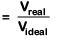∵ Vreal < Videal; Hence Z < 1
If Z < 1, attractive forces are dominant among gaseous molecules and liquefaction of gas will be easy.

Q.3. Given vander Waals constant for NH3, H2 and CO2 are respectively 4.17, 0.244, 1.36 and 3.59, which one of the following gases is most easily liquefied?    (2018)
(a) NH3
(b) H2
(c) O2
(d) CO2
Ans:
a
Critical temperature ∝ vanderwaal constant(a) maximum "a" ⇒ gas with maximum TC ⇒ easiest liquification = NH3

Q.4. The correction factor 'a' to the ideal gas equation corresponds to    (2018)
(a) density of the gas molecules

(b) volume of the gas molecules
(c) electric field present between the gas molecules
(d) forces of attraction between the gas molecules
Ans:
d
Vanderwaal constant (a) ∝ forces of attraction.

Q.5. Equal moles of hydrogen and oxygen gases are placed in a container with a pin-hole through which both can escape. What fraction of the oxygen escapes in the time required for one-half of the hydrogen to escape ?    (2016)
(a) 1/2
(b) 1/8
(c) 1/4
(d) 3/8
Ans:
b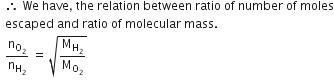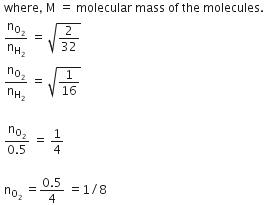Q.6. Equal masses of H2, O2 and CH4 have been taken in a container of volume V at temperature 27° C in identical conditions. The ratio of the volumes of gases H2:O2:CH4 would be :    (2014)
(a) 16 : 1 : 2
(b) 8 : 1 : 2
(c) 8 : 16 : 1
(d) 16 : 8 : 1

Ans: a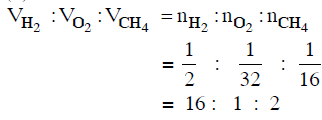Offer running on EduRev: Apply code STAYHOME200 to get INR 200 off on our premium plan EduRev Infinity!

29 docs|52 tests

,

,

,

,

,

,

,

,

,

,

,

,

,

,

,

,

,

,

,

,

,

;